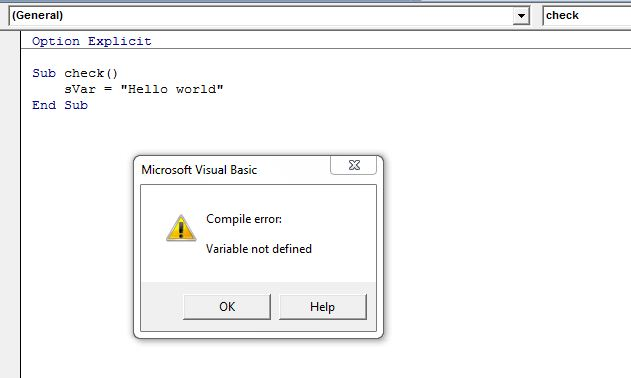# Excel VBA Variables declaration

In this post, you’ll learn Excel VBA variables declaration.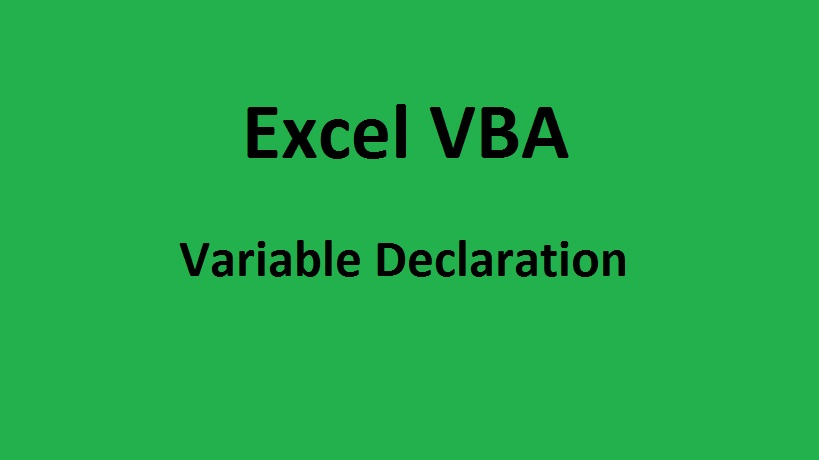## What is Excel VBA Variables?

Excel VBA Variables are used to store different type of data such as integer, String, boolean, double, date, currency etc, in computer memory to use that values in the procedure, module or workbook. The values stored in the variables can be retrieved from computer memory if the user wants to use the values to do further programming before the variables lose their scope. Values stored in the variables are temporary and might be continuously changing based on how it is used in the programming. VBA Programming is almost impossible without using variables in the code.

For e.g. Let us assume that Range A1 in sheet 1 contains some 500 words of text which we call it as String in programming. Whenever you want to use that string you should specify like Thisworkbook.Sheets(1).Range(“A1”).Value. Instead of doing like this you can assign this value to a variable sFirst using the assignment operator.

sFirst =Thisworkbook.Sheets(1).Range(“A1”).Value

Hereafter, when you want to use that string in any part of your programming you can specify it by variable name sfirst.

## Rules to Declare Excel VBA variables

There is a certain programmer should follow when declaring Excel VBA variables. The declared variable name must follow the below Excel VBA variables rules.

• Length of the Excel VBA Variables must be less than 255 characters
• Special characters like (#, \$, %, &, or !) are not acceptable
• Excel VBA Variables must begin with the letter or underscore.
• Excel VBA Variables must not contain period or spaces.
• Excel VBA Variables name must be unique in the module or procedure where it is used.
• Excel VBA Variables must not contain any of the Excel VBA reserved keywords.

Excel VBA reserve some of the keywords for internal use For e.g. Dim, For, Next, Do, Until, And, Or, IsNumeric, If, EndIf, Integer, Double, Date, Select, Case, End Select, etc.,

## How to declare Excel VBA variables?

The recommended way of declaring Excel VBA variables is using Dim Statement as prefix and followed by its data type(Integer, Double, String etc.,). Even Though Dim statement and data type are optional it’s the best programming practices. Variables can also be declared without using a Dim statement and Datatype. If you’re using Dim statement then you’re telling the compiler that you’re declaring a variable but still, the compiler is good enough to determine the variable without the presence of Dim keyword. Data type tells the compiler the type of data that you’re going to store in the variable. If the data type is not provided then compiler uses the default data type called Variant. The variant can store and handle almost all kind of data but it gradually affects the performance of the code.

Syntax

Dim sName As String

Dim- Short for the word Dimension and it helps to determine the variable data type and other information.

sName- It the variable name where the data gets stored.

String- It defines the type of data stored in the variable.

## Excel VBA variables Datatype

Excel VBA variables data type are primarily classified into two types:

1) Numeric data type

2) Non-Numeric Datatype

### 1) Numeric Datatype

The numeric Data type used to deal with numerical formats such as decimal, fraction, Integer etc,. Below are the list of numeric data types, space it allocates to store the value and the range of values it can handle.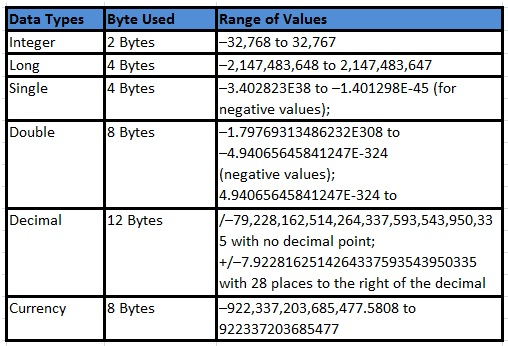#### Declare variable using Integer Data Type

Integer Data type used to store values in the range -32768 to 32767 and allocate 2 Bytes of computer space. When you attempt to store value larger than the integer range then compiler throws an error message “Run time error: Overflow”.

Option Explicit

Sub Exercise1()

Dim aNum As Integer

aNum = 653564

MsgBox aNum, vbOKOnly, “Verify”

End Sub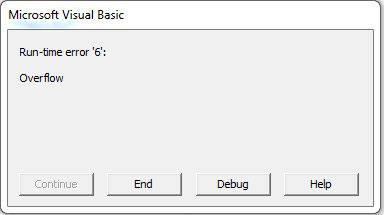### 2) Non-Numeric Data Types

Non Numeric data types are used to deals with non-numerical data such as byte, logical values (true or false), date, string (text) etc. Below is the list of non-numeric data types, space it allocates to store the value and the range of values it can handle.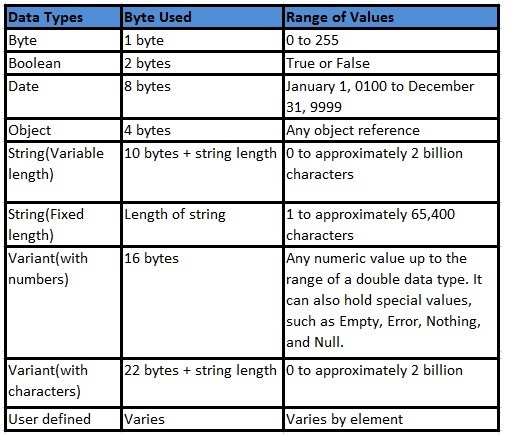#### Declare variable using Boolean Datatype

Boolean data type contains only two values (True or False) and allocates 2 Bytes of computer memory.

Option Explicit

Sub Exercise2()

Dim IsGreater As Boolean

If IsGreater = True Then MsgBox “Boolean Returns True”, vbOKOnly, “Verify”

End Sub

## Option Explicit

Option Explicit command is used to force the programmer to declare all Excel VBA variables before you use. Option Explicit command should be added at the top of the code. It is the best way to force the user to declare the variable and avoid errors due to the misspelling of variables. Before executing the code compiler run from top to bottom of the code to check whether all the variables are declared and if any of the variables is not declared then it pops up an error message stating “Compile Error: Variable Not Defined”.

In the below code, I’ve used an option Explicit command at the top, used variable sVar without declaration and assign the string “Hello World” to it. When I execute with F5 or Run command, VBA throws an Error message and ask the user to declare the variable.